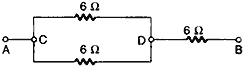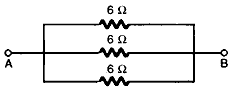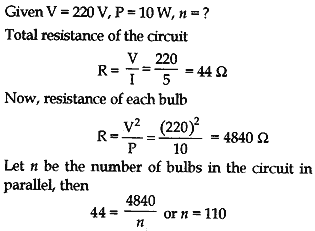Monday , May 23 2022# Electricity: 10th CBSE Science Physics

#### Question: Show how you would connect three resistors, each of resistance 6 Ω , so that the combination has a resistance of (i) 9 Ω , (it) 4 Ω .

Answer: (i) In order to get a resistance of 9 Ω from three resistors, each of resistance 6 Ω, we connect two resistors in parallel and this parallel combination (of resistance 6 Ω, 6 Ω) in series with the resistor as shown below:(ii) We cannot obtain a resistance of 4 Ω by any combination of three 6 Ω resistors. But we can obtain a resistance of 2 Ω, by connecting all of them in parallel as shown below:#### Question: Several electric bulbs designed to be used on a 220 V electric supply line, are rated 10 W. How many lamps can be connected in parallel with each other across the two wires of 220 V line if the maximum allowable current is 5 A?#### Question: A hot plate of an electric oven connected to 220 V line has two resistance coils A and B, each of 24 Ω resistance, which may be used separately, in series, or in parallel. What are the currents in the three cases?

Answer: Given  V = 220 V, R = 24 Ω, I = ?

(i) When each of the coils A or B is connected separately, current through each coil, i.e.,
I = V/R = 220/24 = 9.17 A

(ii) When coils A and B are connected in series, equivalent resistance in the circuit,
Rs = R + R = 24 + 24 = 48 Ω
Therefore, current through the series combination, i.e.,
I = V/R = 220/48 = 4.58 A

(iii) When the coils A and B are connected in parallel, equivalent resistance in the circuit,
1/Rp = 1/24 + 1/24 = 2/24
or Rp = 12 Ω
Therefore, current through the parallel combination, i.e.,
I = V/R = 220/12 = 18.3 A

#### Question: Compare the power used in the 2 Ω resistor in each of the following circuits. (i) a 6 V battery in series with 1 Ω and 2 Ω resistors, and (ii) a 4 V battery in parallel with 12 Ω and 2 Ω resistors.

(i) Since 2 Ω and 1 Ω resistors are in series therefore, equivalent resistance is
Rs = 2 + 1 = 3 Ω
Hence, current through the combinations
I = V/R = 6/3 = 2 A
Hence, power consumed by 2 Ω resistor
Ps = I²R = (2)² × 2 = 8 W

(ii) Since 12 Ω and 2 Ω are in parallel, therefore, potential across 2 Ω resistor is 4 V
Hence, power consumed by 2 Ω resistor is
Pp = V²/R = (4)²/2 = 8 W

Thus, in both cases the power consumed by the 2 Ω resistor is 8 W. Therefore, ratio of power consumed in the two cases is one.

#### Question: Two lamps, one rated 100 W at 220 V, and the other 60 W at 220 V, are connected in parallel to electric mains supply. What current is drawn from the line if the supply voltage is 220 V?

Answer: Given P1 = 100 W, 220 V and
P= 60 W, 220 V
In parallel combination of lamps, the total power consumed is given by
P = P1+ P2
Therefore, we have
P = 100 + 60 = 160 W
Since P = VI, therefore,
I = P/V = 160/220 = 0.727 A

#### Question: Which uses more energy, a 250 W TV set in 1 hr, or a 1200 W toaster in 10 minutes?

Energy consumed by 250 W TV set in 1 h = 250 x 1 = 250 Wh.
Energy consumed by 1200 W toaster in 10 min = 1200 X 1/6 = 200 Wh.
∴ Energy consumed by TV set is more than the energy consumed by toaster in the given timings.